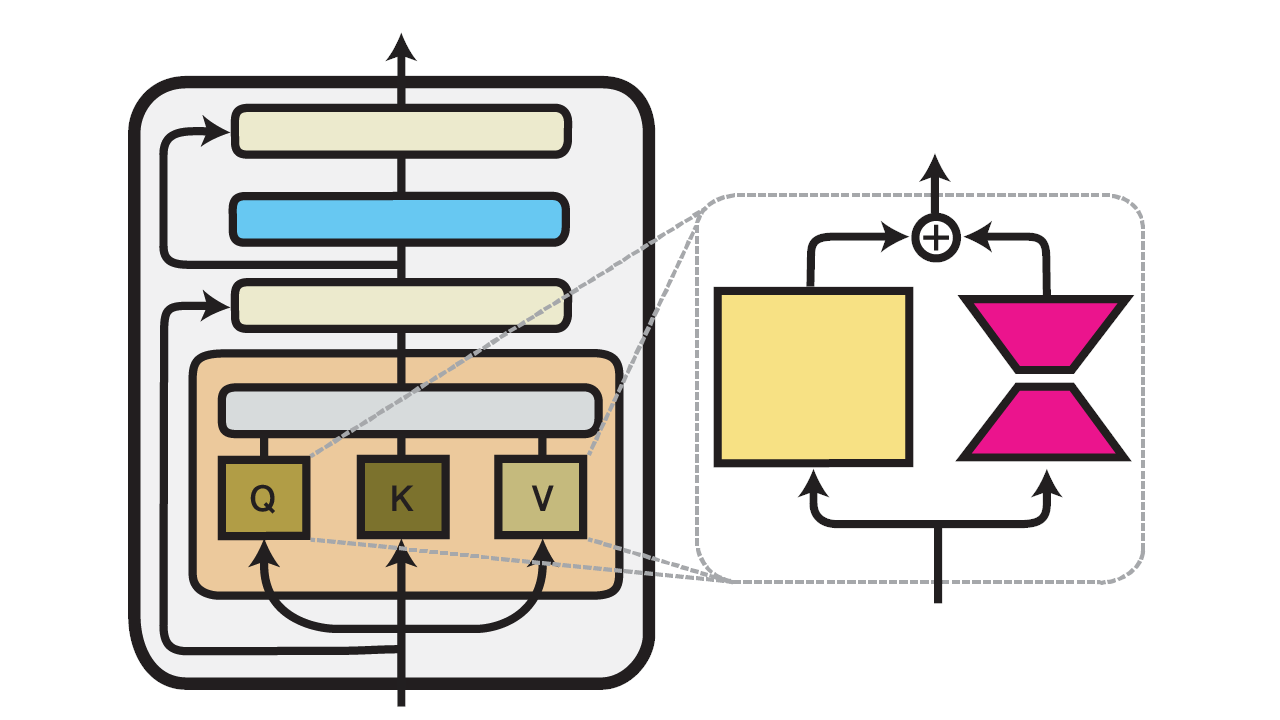# Train a Text Summarizer in 5 Minutes

## Introduction

Have you ever wanted to fine-tune a large language model, but didn't have the time or resources to train it from scratch? In this post, I'll show you how to use adapter-transformers to fine-tune Flan-T5 on the XSum dataset in five minutes. I'll also show you how to use the resulting model to generate summaries for any text.

## What is Fine-Tuning?

Fine-tuning is the process of training a pre-trained model on a new dataset. This usually takes less time than training a model from scratch, but it can still take a long time.

adapter-transformers is a library for fine-tuning transformers, based on HuggingFace's transformers library. It has a number of new features that make it easier to fine-tune transformers, such as LoRA (Low Rank Adaptation), which allow you to reduce the amount of parameters that need to be trained.

## What is LoRA?LoRA works by training two small matrices that are multiplied together to produce a large matrix. This large matrix is then added to the pre-trained model's weights, usually in the attention layers. Adding the matrices is equivalent to adding the results of the matrix multiplication, which is done in training.

## The Flan-T5 Model

Flan-T5 is a series of models by Google that are based on the T5 architecture. They are text-to-text transformers that can be used for a variety of tasks, making them a great starting point for fine-tuning.

In this post, I'll be using flan-t5-base, which is one of the smaller models in the series.

## The XSum Dataset

XSum stands for Extreme Summarization and is a dataset of documents and summaries.

In this post, I'll be using a small subset of the XSum dataset, which contains 1,000 documents and their summaries.

## Environment Setup

I'll be using Google Colab for this post, but you can also run it locally if you have a GPU and the necessary libraries installed.

To install the libraries that aren't installed in Google Colab, you'll have to run the following commands:

## Tokenizing the Dataset

In order to use the Flan-T5 model, we'll have to convert the documents and summaries into tokens that the model can understand.

To do this, we'll use the class from the library. We'll also use a prefix before the documents, which is used by the model to determine what task it should perform. In this case, the prefix is . We have to do this because this is the format the model expects.

We'll be using the library to load the dataset, and we will load a subset of the dataset from each split.

## Preparing the Model

Now that we have the dataset, we can prepare the model for training.

## Preparing the Trainer

Now that we have the model, we can prepare the trainer.

## Training the Model

Now that we have the trainer, we can train the model. This will take a while, especially depending on your hardware.

## Evaluating the Model

Now that we have the trained model, we can evaluate its performance on the test split.

Like I mentioned earlier, we will need to add the matrices.

## Generating Summaries

We will use the validation split of the dataset and compare our summaries with the actual summaries.

## Saving the Model

Now that we have the trained model, we can save it. Mine is saved to .

## Conclusion

Hopefully this tutorial was helpful in showing how to quickly fine-tune a model for a new task using adapters. My source code for this tutorial can be found on Google Colab.